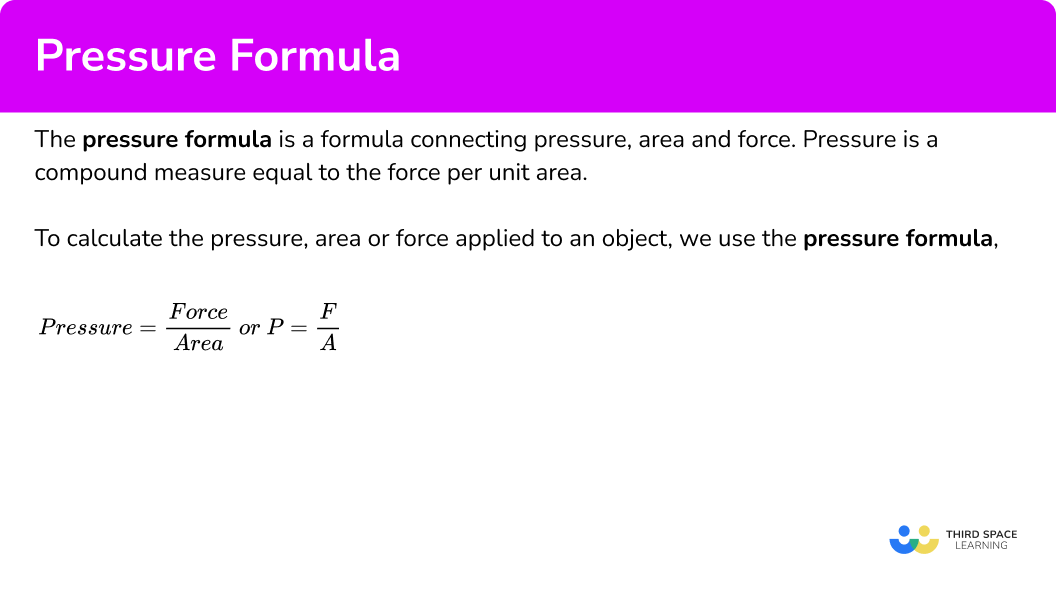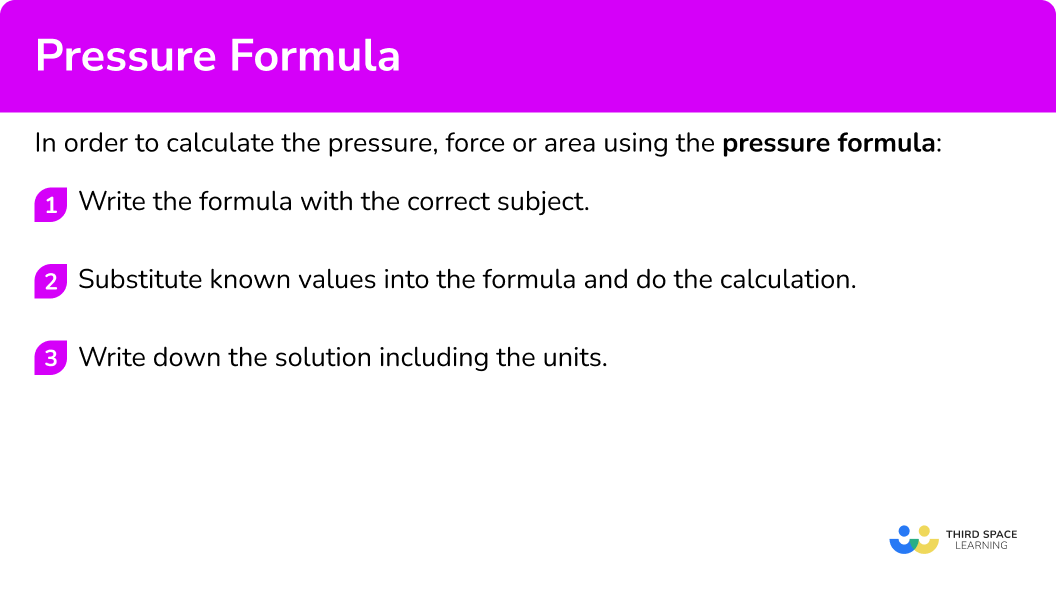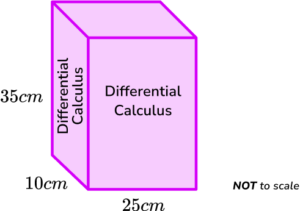# Pressure Formula

Here we will learn about the pressure formula, including how to use the formula.

There are also pressure formula worksheets based on Edexcel, AQA and OCR exam questions, along with further guidance on where to go next if you’re still stuck.

## What is the pressure formula?

The pressure formula is a formula connecting pressure, area and force.

Pressure is a measure of force per unit area. Weight is calculated by multiplying mass by the acceleration of gravity so heavier objects exert more pressure.

To calculate the pressure, area or force applied to an object, we need to use their intrinsic relationship. Pressure is calculated by dividing the amount of force by the area.

The pressure formula (or pressure equation) is therefore

\text{pressure}=\frac{\text{force}}{\text{area}}\text{ or }P=\frac{F}{A} .

Pressure is a compound measure made from force and area. Pressure equals the force per unit area. Pressure is measured using pressure gauges.

Force is the energy attributed to a movement or a physical action. The SI unit for force in Newtons (N).

Area is the amount of a surface in which the force is applied to. The standard unit for area is square metres (m^{2}).

The pressure formula can be used in a formula triangle where it can be adapted to calculate force and area.

The units of force are Newtons (N) and the derived SI units of area are square metres (m^{2}). So the units of pressure are Newtons per square metre (N/m^{2}).

However, the standard unit of pressure is Pascals (Pa). \ 1 Pascal is equal to 1 \ N/m^2 and 100,000 \ Pa = 1 \ Bar (another unit of pressure).

Air pressure (or atmospheric pressure) can change depending on where we are in the world. If we are on a mountain the air pressure is less than when we are at sea level. The air pressure at sea level is approximately 14.7 pounds per square inch or 1.01325 Bar.

### What is the pressure formula?## How to calculate the pressure, force or area using the pressure formula

In order to calculate the pressure, force or area using the pressure formula:

1. Write the formula with the correct subject.
2. Substitute known values into the formula and do the calculation.
3. Write down the solution including the units.

### Explain how to calculate the pressure, force or area using the pressure formula### Related lessons oncompound measures

Pressure formula is part of our series of lessons to support revision on compound measures. You may find it helpful to start with the main compound measures lesson for a summary of what to expect, or use the step by step guides below for further detail on individual topics. Other lessons in this series include:

## Pressure formula examples

### Example 1:  calculating pressure

A force of 600 \ N acts on an area of 20 \ m^{2}. Calculate the pressure.

1. Write the formula with the correct subject.

As P=\frac{F}{A} and we want to calculate the pressure, we do not need to rearrange the formula so we have,

\text{pressure}=\frac{\text{force}}{\text{area}} .

2Substitute known values into the formula and do the calculation.

\text{Pressure}=\frac{\text{force}}{\text{area}}=\frac{600}{20}=30

3Write down the solution including the units.

Pressure is 30 \ N/m^{2}.

The solution can also be written in Pascals, 30 \ Pa.

### Example 2: calculating pressure

A force of 980 \ N acts on an area of 120 \ m^{2}. Calculate the pressure. Give your answer correct to 2 decimal places.

As P=\frac{F}{A} and we want to calculate the pressure, we do not need to rearrange the formula, so we have

\text{pressure}=\frac{\text{force}}{\text{area}} .

\text{Pressure}=\frac{\text{force}}{\text{area}}=\frac{980}{120}=8.1\dot{6}

\text{Pressure}= 8.17 \ N/{m}^{2}\text{ (2dp)} \ (=8.17Pa\text{ (2dp)})

### Example 3: calculating area

A force of 560 \ N acts exerts a pressure of 70 \ N/m^{2}. Calculate the area of the surface that the force is applied to.

As P=\frac{F}{A} and we want to calculate the area, we need to rearrange the formula to make A the subject. We do this by multiplying both sides by A, and then dividing both sides by P, so we have,

\begin{aligned} P&=\frac{F}{A}\\\\ P\times{A}&=\frac{F}{A}\times{A}\\\\ P\times{A}&=F\\\\ P\times{A}\div{P}&=F\div{P}\\\\ A&=\frac{F}{P} \end{aligned}

Alternatively we could use the pressure formula triangle,

\text{Area}=\frac{\text{force}}{\text{pressure}}=\frac{560}{70}=8

\text{Area}=8m^{2}

### Example 4: calculating area

A force of 460 \ N exerts a pressure of 18 \ N/m^{2} onto a flat surface. Calculate the area of the surface that the pressure is being exerted on. Give the answer correct to 3 significant figures.

As P=\frac{F}{A} and we want to calculate the area, we need to rearrange the formula to make A the subject. We do this by multiplying both sides by A, and then dividing both sides by P, so we have,

\begin{aligned} P&=\frac{F}{A}\\\\ P\times{A}&=\frac{F}{A}\times{A}\\\\ P\times{A}&=F\\\\ P\times{A}\div{P}&=F\div{P}\\\\ A&=\frac{F}{P} \end{aligned}

Alternatively we could use the pressure formula triangle,

\text{Area}=\frac{\text{force}}{\text{pressure}}

\text{Area}=\frac{\text{force}}{\text{pressure}}=\frac{460}{18}=25.\dot{5}

\text{Area}=25.6m^{2}\text{ (3sf)}

### Example 5: calculating force

A pressure of 40 \ N/ m^{2} is exerted on an area of 6 \ m^{2}. Calculate the force.

As P=\frac{F}{A} and we want to calculate the force, we need to rearrange the formula to make F the subject. We do this by multiplying both sides by A so we have,

\begin{aligned} P&=\frac{F}{A}\\\\ P\times{A}&=\frac{F}{A}\times{A}\\\\ P\times{A}&=F\\\\ F&=P\times{A} \end{aligned}

Alternatively we could use the pressure formula triangle,

\text{Force}=\text{pressure}\times \text{area}

\text{Force}=\text{pressure}\times \text{area}=40\times 6=240

\text{Force}= 240 \ N

### Example 6: calculating the force

A pressure of 83 \ N/ m^{2} is exerted on an area of 53.2 \ m^{2}. Calculate the force.

As P=\frac{F}{A} and we want to calculate the force, we need to rearrange the formula to make F the subject. We do this by multiplying both sides by A, so we have,

\begin{aligned} P&=\frac{F}{A}\\\\ P\times{A}&=\frac{F}{A}\times{A}\\\\ P\times{A}&=F\\\\ F&=P\times{A} \end{aligned}

Alternatively we could use the pressure formula triangle,

\text{Force}=\text{pressure}\times \text{area}

\text{Force}=\text{pressure}\times \text{area}=83\times 53.2=4415.6

\text{Force}=4415.6 \ N

### Example 7: converting units of area

A pressure of 29 \ N/ m^{2} is exerted on an area of 20000 \ cm^{2}. Calculate the force applied to the area.

As P=\frac{F}{A} and we want to calculate the force, we need to rearrange the formula to make F the subject. We do this by multiplying both sides by A, so we have,

\begin{aligned} P&=\frac{F}{A}\\\\ P\times{A}&=\frac{F}{A}\times{A}\\\\ P\times{A}&=F\\\\ F&=P\times{A} \end{aligned}

Alternatively we could use the pressure formula triangle,

\text{Force}=\text{pressure}\times \text{area}

The area is in square centimeters, so we need to divide by 10,000 to find the area in square metres (1 \ m^{2} = 10,000 \ cm^{2}).

20 \ 000 \div 10 \ 000=2

Now we have area = 2 \ m^{2}, and so

\text{force}=\text{pressure}\times \text{area}=29\times 2=58.

\text{Force}=58 \ N

### Common misconceptions

• Units of measure

We tend to use metric units for area. The derived SI units for area are square metres. Occasionally you might come across other types of units such as imperial units like the square inch.

• Incorrect units

As pressure is a measure of force per unit area (N/m^{2}), the units for force will be given (N), as well as the units for area (m^{2}). Use these to determine the units of your answer. If you are given the pressure in Pascals, 1 \ Pa = 1 \ N/m^2 and so you will need to remember this to determine the units of your solution.

Check that you give the answer in the right form. You may have an answer which needs to be rounded to a specific number of significant figures or decimal places.

• Units of area

To convert centimetres to metres, we divide by 100 as 1 \ m = 100 \ cm. To convert square centimetres to square metres, we divide by 100^{2} =10,000 as 1 \ m^{2} = 10,000 \ cm^{2} .

• Scalar or vector

Area is a scalar quantity as it has size. Force is a vector quantity as it has size and magnitude. Pressure is a scalar quantity too.

### Practice pressure formula questions

1. A force of 600 \ N acts on an area of 30 \ m^{2}. Calculate the pressure.

20 \ N/m^{2}0.2 \ N/m^{2}2 \ N/m^{2}200 \ N/m^{2}\text{Pressure}=\frac{\text{force}}{\text{area}}=\frac{600}{30}=20 \ N/m^{2}

2. A force of 623 \ N acts on an area of 452 \ m^{2}. Calculate the pressure. Give your answer correct to 3 significant figures.

29.3 \ N/m^{2}282000 \ N/m^{2}0.726 \ N/m^{2}1.38 \ N/m^{2}\text{Pressure}=\frac{\text{force}}{\text{area}}=\frac{623}{452}=1.3783…=1.38 \ N/{m}^{2}\text{ (3sf)}

3. A force of 800 \ N acts exerts a pressure of 20 \ N/m^{2}. Calculate the area that the force is applied to.

400 \ m^{2}40 \ m^{2}30 \ m^{2}16 \ m^{2}\text{Area}=\frac{\text{force}}{\text{pressure}}=\frac{800}{20}=40 \ m^2

4. A force of 463 \ N exerts a pressure of 9.8 \ N/m^{2}. Calculate the area that the force is applied to, correct to 2 decimal places.

4537.40 \ m^{2}47.24 \ m^{2}0.02 \ m^{2}4.82 \ m^{2}\text{Area}=\frac{\text{force}}{\text{pressure}}=\frac{463}{9.8}=47.24489796…=47.24 \ {m}^2\text{ (2dp)}

5. A pressure of 25 \ N/m^2 is exerted on an area of 6 \ m^{2}. Calculate the force.

4.2 \ N0.69 \ N150 \ N900 \ N\text{Force}=\text{pressure}\times \text{area}=25\times 6=150 \ N

6. A pressure of 89 \ N/m^2 is exerted on an area of 17.3 \ m^{2}. Calculate the force. State the units in your answer.

1539.7 \ g15.39.7 \ kg1539.7 \ Pa1539.7 \ N\text{Force}=\text{pressure}\times\text{area}=89\times{17.3}=1539.7 \ N

### Pressure formula GCSE questions

\text{Pressure}=\frac{\text{Force}}{\text{Area}}

1. A tank contains sea water.

Work out the force when the pressure is 72 \ N/m^2 and the area of the base is 8 \ m^{2}.

A B C D

(1 mark)

9 \ N

(1)

2. Work out the area when the pressure is 96 \ N/m^2 and the force is 4800 \ N.

(2 marks)

4800\div{96}

(1)

50 \ m^2

(1)

3. A book about Differential Calculus is modelled as a cuboid below.The dimensions of the book are 35 \ cm \ (H), \ 10 \ cm \ (D) and 25 \ cm \ (W).

The downwards force of book on the shelf is 30 \ N.

Calculate the pressure the book exerts on the shelf.

(4 marks)

\text{Area}=10\times 25=250

(1)

\text{Area}=250\div 100^2=0.025

(1)

30\div 0.025

(1)

1200 \ N/{m}^{2}\textbf{ or }1200 \ Pa\textbf{ or }0.012 \ Bar

(1)

## Learning checklist

You have now learned how to:

• Convert between related compound units (pressure) in numerical and algebraic contexts

## Beyond GCSE

Here are a few more facts about pressure,

• The density of a gas is equal to the mass divided by its volume. For example, at 0°C, the density of the gas Oxygen is 1.429 \ kg/m^{3}.
• Absolute pressure is the pressure that exists in a space with no matter, such as in a perfect vacuum.
• Partial pressure is the pressure exerted within a mixture by an individual gas (such as the partial pressure of nitrogen gas in the air at sea level is 593 \ mmHg or 79058.76 \ Pa ).
• In thermodynamics, the ideal gas law states that the gas constant R is equal to the pressure times the volume of a gas, divided by the number of moles and temperature of the gas. This constant of proportionality R = 8.3145 \ J/mol/K (Joules per Mole per Kelvin) where Joules are the SI unit of energy, Moles are the SI unit for the amount of a substance, and Kelvin is the SI unit of temperature.
• In fluid mechanics, Hydrostatic pressure (fluid pressure, or water pressure) is the pressure in a liquid at a given depth. The hydrostatic pressure can be calculated using the formula P=ρgh where P represents the pressure, ρ the greek letter rho is the density of the liquid, g is acceleration due to gravity for the height h (or depth) of the liquid. If the density of the liquid and the acceleration due to gravity are constant, the pressure due to the fluid column is directly proportional to the height of the fluid column.
• Bernoulli’s principle states that the speed of a fluid increases when static pressure decreases. Bernoulli’s principle allows us to calculate the total pressure of a fluid or gas mixture.

## Still stuck?

Prepare your KS4 students for maths GCSEs success with Third Space Learning. Weekly online one to one GCSE maths revision lessons delivered by expert maths tutors.

Find out more about our GCSE maths tuition programme.